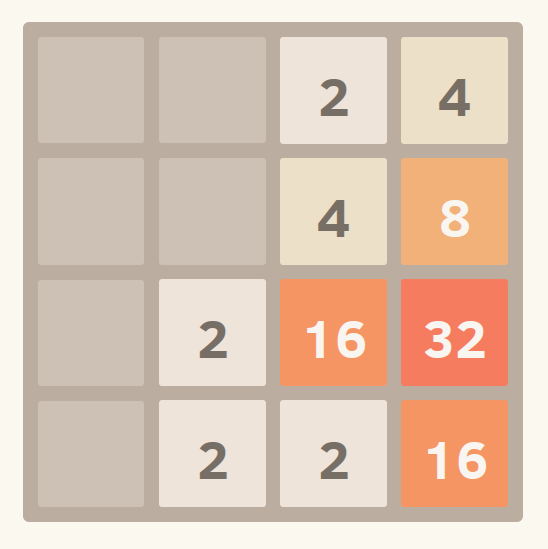# 4096 I

Logic Level 2

Chris decided to code a game called 4096 which he believes is the next big thing. The game is relatively simple. You have a $4\times 4$ grid that contains integers that are all powers of 2. Tiles slide as far as possible in the chosen direction until they are stopped by either another tile or the edge of the grid. If two tiles of the same number collide while moving, they will merge into a tile with the total value of the two tiles that collided. The resulting tile cannot merge with another tile again in the same move.

The possible moves are $L,R,U,D$ which means swipe to the left, right, up and down respectively.wikipedia

After sweeping in the order : $U,L,D,L,U,R,D$, what is the largest number found in the grid?

Clarification: No new tiles are added after each move.

×